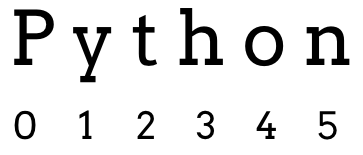# 6.5. Loop Through a String¶

The `range` command assigns the loop variable a new integer value each time the loop repeats. What if we want to assign the variable something other than whole numbers?

## 6.5.1. Characters Instead of Integers¶

Recall that a string is a series of characters enclosed in quotes, like `'Hello, World!'` or `"Python"`. Just like `range` assigns a new integer to the loop variable, we can set up a `for` loop to assign different characters from a string.

Run the following program to see how this works:

Some points to notice:

1. In the `for` statement, a string (or a variable containing a string) replaces `range`.

2. The first time the loop runs, the loop variable gets assigned the first character in the string (`character = 'H'` or `char = 'T'`).

3. Each time the loop repeats, the variable holds the next character in the string.

4. Unlike `range(n)`, which does NOT assign the value of `n` to the loop variable, the last character in the string IS used.

5. Once Python reaches the end of the string, the loop ends.

## 6.5.2. Characters Another Way¶

Take a look at the following code and its output:

Example

 ```1 2 3 4 5 6``` ```word = 'Python' print(word) print(word) print(word) print(word) ```

Console Output

```Python
P
y
n
```

Notice how lines 4 - 6 each print a single character from the string. `print(word)` displays only the first letter (`P`). `word` prints the second character, and `word` prints the last.

We will explore strings in more detail in a later chapter. For now, we just need to recognize that every character in a string has an index. An index is a number that tells us the location of a character in the string.

Like `range`, the index values of a string start counting at `0`.The index values of a string.

Indexes provide a different way to loop through a string:

Example

Some items to note:

1. By using `range(6)`, the `index` variable takes values from 0 - 5.

2. `word[index]` refers to the character in the string at position `index`.

3. As written, the loop only displays characters up to index 5.

• Try replacing the string with a longer one.

4. If we replace the string with a shorter one, we get an error! For `word` to work, there must be a character at index 5.

5. To prevent “index out of range” errors in this loop, we should NOT use a specific number inside `range`.

6. Replace `range(6)` with `range(len(word))`. `len(word)` always returns the length of the current string, so changing that string will not break the loop.

Question

If `phrase = 'Code for fun'`, then `phrase` evaluates to:

1. "o"
2. "d"
3. "for"
4. "fun"

Question

What will be printed in the THIRD iteration of the loop:

 ```1 2``` ```for char in 'ABCDEFGHIJ': print(char) ```
1. "ABC"
2. "B"
3. "C"
4. "D"